Connect with us

# The Principles of Discounted Cash Flow and Its MeasurementPublished

on

Let’s start out by identifying a number of key conceptual premises of “Discount Cash Flow (DCF)”:

0. means period zero, or the starting point of the project.
1. means one year from the start of the project.
2. means two years from the start of the project, and so forth.

A simple example is:

0. (\$1,000) cash outflow (parentheses)
1. \$1,020 cash inflow (no parentheses)

Clearly this is not a particularly attractive investment, since if the money were put in a local bank, the return might be 5 percent:

0. (\$1,000)
1. \$1,050

Therefore, we have already established two basic principles of Discounted Cash Flow:

1. It is measuring profitability
2. Risk issues are incorporated

Profit \$ = Interest \$

Now consider the following financial relationship:

0. (\$1,000)
1. \$1,200

If this were the purchase of a stock and its sale one year later, the profit would be \$200. If this were a bank loan, where you borrowed \$1,000 and repaid \$1,200 after a year, the interest would obviously be \$200.

These are the same concept; the only differences are semantic…..

ROI % = Interest %

The return on investment (ROI) on the stock purchase and sale would be 20 percent. The interest rate on the loan would also be 20 percent, as follows: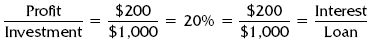The interest rate is the annual fee that the banker charges for the loan. The ROI is the annual fee (ROI requirement) that we impose on an investment.

Time Value of Money Concept

Consider the following:

0. (\$1,000)
2. \$1,200

While the dollar amount of interest and profit remained at \$200, the ROI and interest rate declined to approximately 10 percent. Therefore, ROI and interest rates are annual concepts. Discounted cash flow is based upon the time value of money concept. What this means is that we not only value how much cash flow is generated, but also are very concerned with when it is received—its timing. Sooner is better.

Principal First

The following two investments are not the same:

A                 B
0. (\$1,000)     (\$1,000)
1. \$1,200        \$ 200
2. \$ 200

Note: he ROI in alternative B is negative. In fact, the figures for alternative B show a loss of \$600. For an ROI to be achieved at all, a return of the investment itself must come first. In the case of a loan, the banker wants the principal to be repaid before the interest is recognized.

Discounted Cash Flow Measures

The basic premises of discounted cash flow have now been identified.

1. It is measuring profitability.
2. Risk issues are incorporated.
3. Profit \$ = Interest \$.
4. ROI % = Interest %.
5. It is an annual concept.
6. Principal must be returned first.

While these key points are all interdependent, the critical one is that interest rate and ROI are calculated in the same way. The basis of discounted cash flow technique is to use present value tables to calculate ROI. Let’s have a look at the following analysis: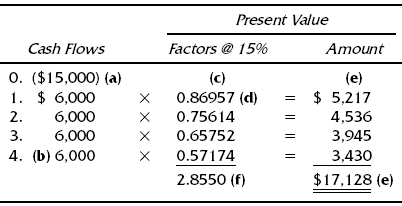Each lettered item in the analysis is addressed individually.

(a) The company is considering an investment of \$15,000. It wants to buy a machine that will help it to increase revenue and the resulting cash flow by enabling it to add more features and benefits to its products.

(b) The company estimates that this opportunity will benefit it for four years. This might be determined by the physical life of the machine, the market life of the features and benefits the machine will make possible, or the market life of the product line itself. Alternatively, the company’s forecasting horizon may be four years. The time period involved may very well be determined by the company’s comfort level.

(c) The company has determined that the minimum required return on investment for this particular opportunity is 15 percent. The company may or may not use the same required level of ROI for all projects. Some companies call the required ROI the hurdle rate (that must be ‘‘jumped over’’ by the project). The hurdle rate may or may not be the same as the company’s cost of capital. There are many different versions of this terminology, so be careful. ROI and interest rate are the same. Therefore, 15 percent is also the interest rate. The annual fee that the company will ‘‘charge’’ the project for the use of the company’s money is the equivalent of the annual fee that the bank charges for a loan. The 15 percent is also the time value of money (TVOM) of that annual fee. In terms of discounted cash flow technique, the 15 percent is called the factor. ROI, interest rate, TVOM, and factor are four synonymous terms.

(d) These decimals are the present value factors. The decimal given for each year is 15 percent less than the decimal given for the previous year. These factors can all be found in an annuity analysis.

(e) The annual cash flow forecast is multiplied by the present value factors. The results are the present value amounts. Through this procedure, each year’s forecast cash inflow is penalized by 15 percent times the number of years the company will have to wait for that cash inflow. We are in fact ‘‘discounting the cash flows’’—hence the name of this technique. The four cash inflows add up to \$17,128. This is called the present value of the cash inflows. It is, in fact, the value of the deal. If this company invested \$17,128 and achieved cash inflows of \$6,000 per year for four years, the ROI would be exactly 15 percent. In this example, the machine cost less than \$17,128. Therefore, the return on investment is greater than 15 percent.

The specific measures of profitability that can be used to evaluate this investment are:

1. Net Present Value (NVP)
2. Profitability Index (PI)
3. Internal Rate of Return (IRR)

Net Present Value

The net present value is a dollar amount. It is calculated as follows: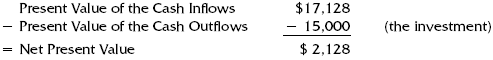A net present value (NPV) that is a positive amount means that the actual return on investment exceeds the target rate, in this case 15 percent. An NPV that is negative means that the actual ROI is below the target. If the NPV is equal to zero, the ROI percentage used to do the calculation is the actual ROI.

Profitability Index

The profitability index (PI) is the comparison of the actual ROI to the target ROI. Its calculation is: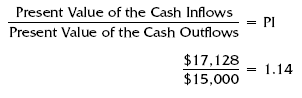A profitability index greater than 1.0 means that the actual ROI exceeds the target. A profitability index lower than 1.0 means that the actual ROI is below the target. If the PI is exactly 1.0, the ROI percentage used in the calculation is the actual ROI.

In this example, we now know with certainty that the actual return on investment exceeds 15 percent, as the NPV is a positive amount and the PI exceeds 1.0. The NPV and the PI will never give conflicting signals, and there will never be conflicting signals between the NPV and PI and the actual return on investment.

Within the realm of normal business forecasting, such conflicting signals are impossible.

Notice (f) on the previous analysis that we repeatedly multiplied \$6,000 times each individual annual factor. As an alter native, we can add up the four annual factors, giving us 2.855, and multiply this number by the annual cash flow.

Except for differences introduced by rounding, doing one summary multiplication will give the same result as the individual calculations. The sum of the annual factors is called an annuity factor. Annuity factors can be used only when the annual cash inflows are the same amount; when cash inflows differ, the present value factors for the individual years must be used. The annuity factors for different time periods and interest rates have been calculated.

Internal Rate of Return

Consider the following formula:

PVCO = PVCI Factor (%, yrs)

This is the formula for the internal rate of return, which is the actual ROI based upon discounted cash flow technique. This formula is in all computer software dealing with this technique. In words, it reads:

The present value of the cash outflows (investment) will be equal to the present value of the cash inflows when multiplied by the correct factor.

The correct factor means the factor corresponding to the right percentage and the right number of years. We now have a critical formula in which three of the four parameters are known. Returning to the example: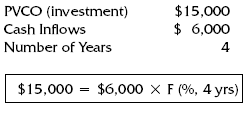Solving for the factor algebraically, we get \$15,000/\$6,000, or 2.5.

Now, if we search in an annuity analysis, we will find on a certain year the factor 2.5 notice that for 4 years, at 20 percent the factor is 2.588, and at 24 percent the factor is 2.404. Therefore, the actual return on investment is between 20 percent and 24 percent. In fact, it is approximately 21 percent. Remember that an annuity analysis table can be used only if the annual cash inflows are the same amount. If the annual cash inflows are different, the method described here will work, but since the formula will include each cash flow amount multiplied by the present value factor for that amount, more trial and error and number crunching will be required.

Payback Period

The payback period is the amount of time that it takes for the cash inflows from the investment to be exactly equal to the investment. It is a cash flow breakeven. While it is not a measure of profitability, it is a measure of risk. Consideration of the payback period is especially valuable for companies with tight cash flow situations. While the company does not want to shut off investment completely, it needs to focus on those opportunities that will have the greatest positive effect on its cash position. For the opportunity that has been analyzed here, the payback period is calculated as follows: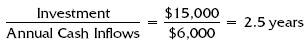This measure is sometimes the only tool that companies use to evaluate an opportunity. The problem with it is that, in addition to the fact that it is not a measure of profitability, it treats all cash flows within the payback period equally, without regard to their time value, and it ignores all cash flows after the payback period.

Are you looking for easy accounting tutorial? Established since 2007, Accounting-Financial-Tax.com hosts more than 1300 articles (still growing), and has helped millions accounting student, teacher, junior accountants and small business owners, worldwide.